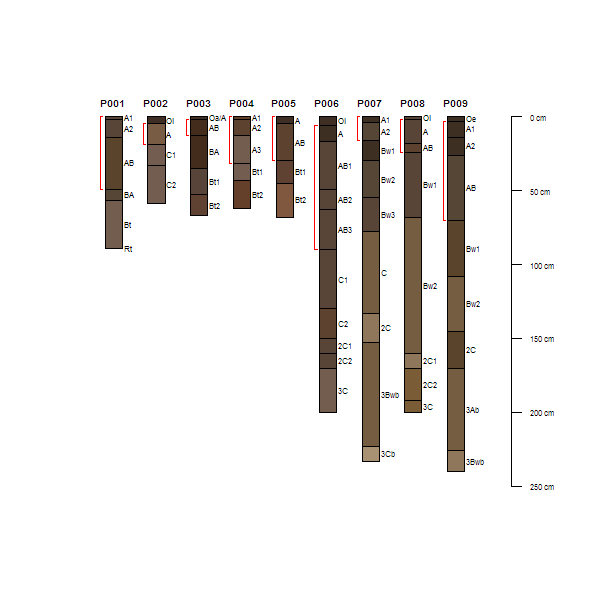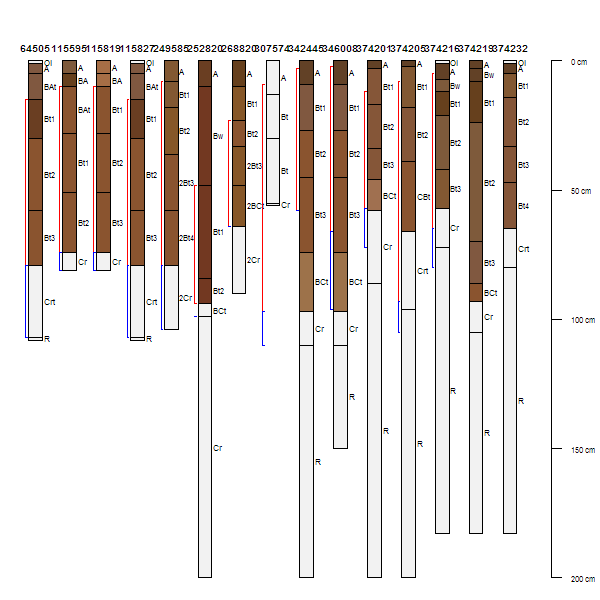addBracket {aqp} R Documentation

## Add Depth Brackets

### Description

Add depth-wise brackets to an existing plot of a SoilProfileCollection object.

### Usage

```addBracket(top, bottom=NULL, idx=NULL, label=NULL, label.cex=0.75,
tick.length = 0.05, arrow.length = 0.05, offset = -0.3,
missing.bottom.depth = 25,
...)
```

### Arguments

 `top` numeric vector of bracket top depths `bottom` numeric vector of bracket bottom depths `idx` optional integer index, associating bracket with profile `label` optional vector of labels for brackets `label.cex` scaling factor for label font `tick.length` length of bracket "tick" mark `arrow.length` length of arrowhead `offset` numeric, length of left-hand offset from each profile `missing.bottom.depth` distance (in depth units) to extend brackets that are missing a lower depth `...` further arguments passed on to `segments` or `arrows`

### Details

The optional argument `idx` can be used to manually specify which profile a given bracket will be associated with. When `idx` is NULL, an integer sequence associated with plotting order (via `plotSPC`) is used. See examples below.

### Note

This is a 'low-level' plotting function: you must first plot a `SoilProfileCollection` object before using this function.

### Author(s)

D.E. Beaudette

`plotSPC`

### Examples

``````# sample data
data(sp1)

# add color vector
sp1\$soil_color <- with(sp1, munsell2rgb(hue, value, chroma))

# promote to SoilProfileCollection
depths(sp1) <- id ~ top + bottom

# plot profiles
plot(sp1)

# extract top/bottom depths associated with all A horizons
f <- function(i) {
h <- horizons(i)
idx <- grep('^A', h\$name)
c(min(h\$top[idx]), max(h\$bottom[idx], na.rm=TRUE))
}

# apply function to sp1, result is a list
a <- profileApply(sp1, f, simplify=FALSE)
# convert list into matrix
a <- do.call('rbind', a)

# plot
plot(sp1)
# annotate with brackets
# note that plotting order is derived from the call to `plot(sp1)`
addBracket(a[, 1], a[, 2], col='red')
````````````# more interesting example using diagnostic horizons
if(require(soilDB)) {
# load some sample data with diagnostic horizons
data(loafercreek)

# extract first 15 profiles
x <- loafercreek[1:15, ]
s <- site(x)

# plot
par(mar=c(0,0,0,0))
plot(x, name='hzname', id.style='top')

# add brackets describing the argillic horizon
addDiagnosticBracket(x, 'argillic horizon', col='red')
# add brackets describing paralithic contact
addDiagnosticBracket(x, 'paralithic contact', col='blue')
}
``````[Package aqp version 1.9.1 Index]